# Viète theorem

on roots

A theorem which establishes relations between the roots and the coefficients of a polynomial. Letbe a polynomial of degreewith coefficients from some field and with leading coefficient 1. The polynomialsplits over a field containing all the roots of(e.g. over the splitting field of, cf. Splitting field of a polynomial) into linear factors:where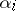are the roots of,. Viète's theorem asserts that the following relations (Viète's formulas) hold: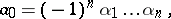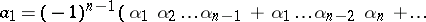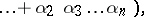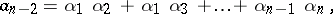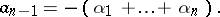F. Viète  proved this relation for all, but for positive roots only; the general form of Viète's theorem was established by A. Girard .